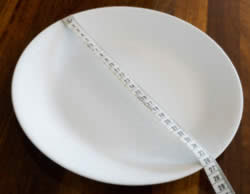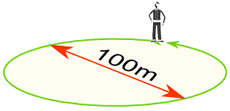## Reading 2017

### Maths

As part of our dome project we have been learning about pi - watch the following at the Wonderopolis
Finding Pi Yourself
Draw a circle, or use something circular like a plate.
Measure around the edge (the circumference):I got 82 cm
Measure across the circle (the diameter):I got 26 cm
Divide:
82 cm / 26 cm = 3.1538...
That is pretty close to π. Maybe if I measured more accurately?

## Digits

In fact π is approximately equal to:
3.14159265358979323846…
The digits go on and on with no pattern. π has been calculated to over two quadrillion decimal places and still there is no pattern to the digits

### Example: You walk around a circle which has a diameter of 100 m, how far have you walked?Distance walked = Circumference = π × 100 m = 314.159... m = 314 m (to the nearest m)

## Approximation

A quick and easy approximation for π is 22/7
22/7 = 3.1428571...
But as you can see, 22/7 is not exactly right. In fact π is not equal to the ratio of any two numbers, which makes it an irrational number.

A better approximation (but still not exact) is:
355/113 = 3.1415929...
(think "113355", then divide the "355" by the "113")

## Remembering The Digits

I usually just remember "3.14159", but you can also count the letters of:
"May I have a large container of butter today"
3 1 4 1 5 9 2 6 5

## To 100 Decimal Places

Here is π with the first 100 decimal places:
 3.1415926535897932384626433832795028841971693993751058209749445923078164062862089986280348253421170679...

### Calculating Pi Yourself

There are many special methods used to calculate π and here is one you can try yourself: it is called the Nilakantha series (after an Indian mathematician who lived in the years 1444–1544).
It goes on for ever and has this pattern:
3 + 42×3×4 − 44×5×6 + 46×7×8 − 48×9×10 + ...
(Notice the + and  pattern, and also the pattern of numbers below the lines.)
It gives these results:
TermResult (to 12 decimals)
13
23.166666666667
33.133333333333
43.145238095238
...... etc! ...
Get a calculator (or use a spreadsheet) and see if you can get better results.

### Pi Day

Pi Day is celebrated on March 14. March is the 3rd month, so it looks like 3/14

Place Value - Parody Shake it Off

Adding Fractions
Follow these steps

Subtracting Fractions

Multiplying Fractions

Ratios

Proportions

Fractions, percentages and ratios and proportions in everyday use - watch the videos and then choose a level.

Addition of Decimals

Calculating Percentages
Follow these steps
What are percentages? and Finding a percent of a number.

Algebra Manipulatives

Maths Learning in Hoiho Class - Sharing the knowledge.

Percentage Game
Have some fun using percentages, decimals and fractions when you plan a park. Follow the instructions at the following link.

Factorising

Order of Operations

Algebra
Introduction

Measurement
Converting Units

Division
Follow these steps and work your way through the questions

Length - We are learning to
• describe a method to measure the length of circular objects
• measure length using metres, centimeters and millimeters
• calculate the circumference of a circle from a measurement of diameter

Our focus this week is to use thermometers to measure temperature in degrees Celsius and investigate factors that influence temperatures
Visit the following links this week to help you understand our learning outcomes

Negative Numbers
click on the link and play the game

Mathletics is now working.  Please select Integers or Decimals if this is your groups learning focus.

Watch these you tube clips on integers (negative numbers)

Check out this catchy song to help you understand place value and decimals.

http://www.mathsisfun.com/measure/thermometer.html

#### 3 comments:

1.I LOVE MATH!!!!!!!

2.Math is fun but also frustrating!!!

3.Finally Fractions!